# GRE Subject Test: Chemistry : Equilibrium Constant

## Example Questions

### Example Question #3 : Equilibrium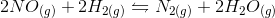In the above reaction, by what factor would the reaction quotient change if the concentration of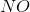were doubled?No changeExplanation:

For a general chemical equation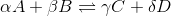, where A, B, C, and D are elements and the Greek letters are their coefficients, we have the reaction quotient equation: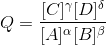We can find the reaction quotient equation for our reaction by substituting the variables.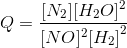Notice that the concentration ofis in the denominator and is squared, so doubling the concentration ofchanges the reaction quotient by a factor of one-fourth.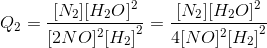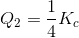### All GRE Subject Test: Chemistry Resources